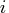Previous Page | Next Page

 Language Reference

## SORTNDX Call

creates an index to reorder a matrix by specified columns

CALL SORTNDX( index, matrix, by<, descend >)

The SORTNDX call returns the following value:

index
is a vector such that index[] is the row index of theth element of matrix when sorted according to by and descend. Consequently, matrix[index, ] is the sorted matrix.
The inputs to the SORTNDX call are as follows:

matrix
is the input matrix, which is not modified by the call.
by
is either a numeric matrix of column numbers, or a character matrix containing the names of columns corresponding to column labels assigned to matrix by a MATTRIB statement or READ statement.
descend
is an optional matrix, indicating which columns, if any, should be descending order. Any by columns not specified as descending will be ascending. If descend = by, then all by columns will be descending; if descend is skipped or is a null matrix, then all by columns will be ascending.

The SORTNDX call can be used to process the rows of a matrix in different sorted order, without having to actually modify it.

For example, the following statements result in the output shown:

```
m = { 1 1 0,
2 0 0,
1 3 1,
2 2 2 };
call SORTNDX( ndx, m, {1 3}, {3} );
```

```
NDX

3
1
4
2
```

The matrix can be physically sorted with the SORT call), as follows:

```
call SORTNDX( ndx, m, by );
m = m[ndx,];
```

The SORTNDX call can be used with the UNIQUEBY function to extract the unique combinations of values in the by columns.Previous Page | Next Page | Top of Page